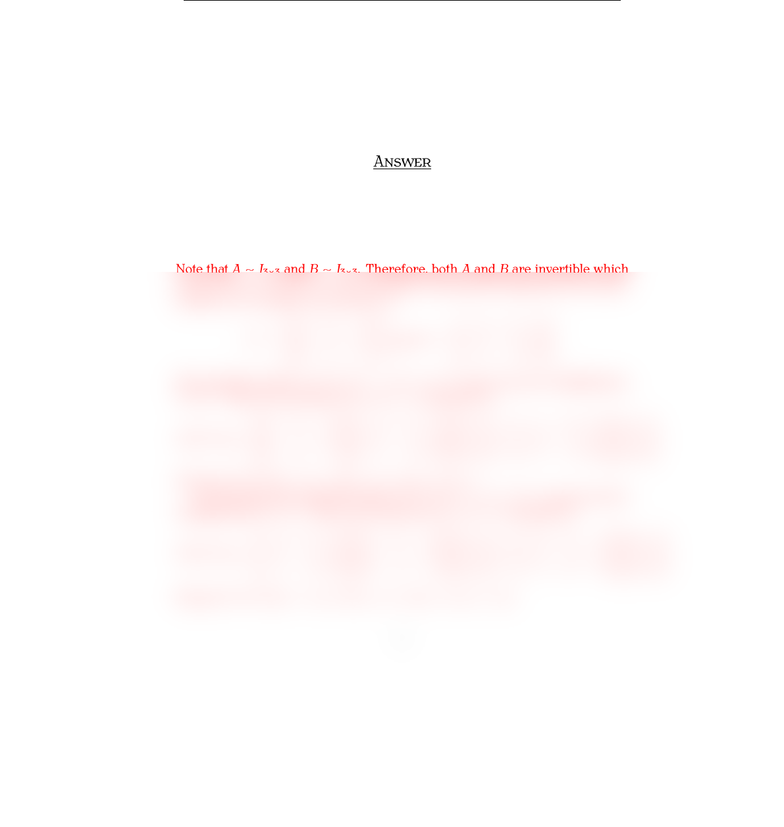# Quiz7mt210SPR11Ans

30 views1 pagesMT Q 
İ S. Y
M , 
Surname, Name:
Q . §. C  I M
Let T:R3ÏR3be the linear transformation deﬁned by
(x1, x2, x3)(x1+x2+x3, x2+x3, x3).
Let S:R3ÏR3be the linear transformation deﬁned by
(x1, x2, x3)(x3, x2+x3, x1+x2+x3).
(A) Is STinvertible? If it is, ﬁnd the formula for (ST)1.
(B) Is TSinvertible? If it is, ﬁnd the formula for (TS)1.
A
(A) The standard matrices Aand Bof Tand S, respectively, are
A=
111
011
001
and B=
0 0 1
0 1 1
1 1 1
.
Note that AI3×3and BI3×3. Therefore, both Aand Bare invertible which
means that STand TSare invertible. By using the algorithm for inverse
matrices, we calculate the inverses as
A1=
11 0
0 1 1
0 0 1
and B1=
01 1
1 1 0
1 0 0
.
The standard matrix of (ST)1=T1S1is given by the multiplication
A1B1. Thus, the formula for (ST)1is obtained as
(ST)1(x) =
11 0
0 1 1
0 0 1
01 1
1 1 0
1 0 0
x1
x2
x3
=
12 1
2 1 0
1 0 0
x1
x2
x3
.
We get (ST)1(x) = (x12x2+x3,2x1+x2, x1).
(B) Similarly, the standard matrix of (TS)1=S1T1is given by the
multiplication B1A1. Thus, the formula for (ST)1is obtained as
(TS)1(x) =
01 1
1 1 0
1 0 0
11 0
0 1 1
0 0 1
x1
x2
x3
=
01 2
1 2 1
11 0
x1
x2
x3
.
We get (TS)1(x) = (x2+ 2x3,x1+ 2x2x3, x1x2).
1
Unlock document

This preview shows half of the first page of the document.
Unlock all 1 pages and 3 million more documents.